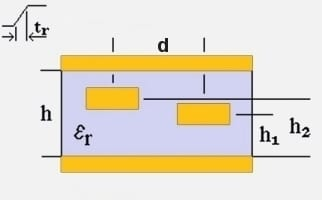Crosstalk

## Stripline Crosstalk Calculator### Inputs

Source Rise Time
tr
ns
Source Voltage
v
Volts
Length of Parallel Traces
l
Substrate Height
h
Trace 1 Height
h1
Trace 2 Height
h2
Trace Pitch
d
Substrate Dielectric
εr

### Output ⊕

Cross Talk Coeff:
-4.75 dB
Coupled Voltage:
0.579 Volts

View Notes

#### Calculation Notes

Backward crosstalk is calculated by this tool, the other type is forward crosstalk which is less dominant. Crosstalk betweem traces is the (normally unwanted) coupled energy.

Factors that influence the crosstalk calculation include:-

• Source rise time (tr)
• Source voltage (v)
• Length of parallel traces (l)
• Substrate height (h)
• Trace heights (h1, h2)
• Trace pitch (d)
• Substrate dielectric (εr)

T_{RT} \approx 1.017\sqrt{\varepsilon _{r}}\times l \times 2  (round trip propagation time)

d_{eff}=\sqrt{d^{2}+\left (h_{2}-h_{1} \right )^{2}}  (effective height above plane)

h_{1eff}=\frac{h_{1}\times \left ( h-h_{1} \right )}{h_{1}+ \left ( h- h_{1} \right )}  (effective h1 height)

h_{2eff}=\frac{h_{2}\times \left ( h-h_{2} \right )}{h_{2}+ \left ( h- h_{2} \right )}  (effective h2 height)

if

\frac{T_{RT}}{t_{r}}\leq 1

then

CT_{dB} \approx 20\log \Bigg ( \frac{1}{1+\left ( \frac{ {d_{eff}}^{2}}{h_{1eff}\times h_{2eff} } \right )}\times \frac{T_{RT}}{t_{r}} \Bigg )

V_{crosstalk} \approx v\times \frac{1}{1+\left ( \frac{{d_{eff}}^{2}}{h_{1eff}\times h_{2eff} } \right )}\times \frac{T_{RT}}{t_{r}}

else

CT_{dB} \approx 20\log \Bigg ( \frac{1}{1+\left ( \frac{ {d_{eff}}^{2}}{ h_{1eff}\times h_{2eff}} \right )^{2}}\Bigg )

V_{crosstalk} \approx v\times \frac{1}{1+\left ( \frac{ {d_{eff}}^{2}}{ h_{1eff}\times h_{2eff}} \right )^{2}}

References:
• High-Speed Digital Design (ISBN 0-13-395724-1)
• Signal Integrity Issues and Printed Circuit Board Design (ISBN 0-13-335947-6)
• Douglas Brooks, "Crosstalk, Part 1: The Conversation We Wish Would Stop!", 1997

Disclaimer: The information and this tool are provided with no liability of any kind whatsoever, use at your own risk.